## Time constraint method

Time constraint method

Types of time constraint method

The time constraint method specifies the time relationship between this process and previous process.

#### (End-Start)

Defines the minimum amount of time separating the end of the previous process and the start of the current process.
Used to strictly separate the previous process and the current process.

#### (Start-Start)

Defines the minimum amount of time separating the start of the previous process and the start of the current process.
Used to allow the previous process and the current process overlap.

#### (Start-Start,End-End)

Defines the minimum amount of time separating the start of the previous process and the start of the current process, as well as the minimum amount of time separating the end of the previous process and the end of the current process.
Used to make the previous process and the current process overlap, while ensuring that the current process does not end before the previous process.

#### (End-Each-Start)

Defines "EES" to define the minimum amount of time separating arbitrary times of the previous process and the production start times of the current process.
If the operation of the current process is split, the move time defines the minimum amount of time separating arbitrary times of the previous process and the production start times of the current process according to the production quantity of each split operation.
If the operation of the current process is not split, then the assignment will be the same as when you specified ES as the time constraint method.

#### (End-Start-Each)

Defines "ESE" to define the minimum amount of time separating the production end times of the previous process and arbitrary times of the current process.
If the operation of the previous process is split, the move time defines the minimum amount of time separating the production end times of the previous process and arbitrary times of the current process according to the production quantity of each split operation.
If the operation of the current process is not split, then the assignment will be the same as when you specified ES as the time constraint method.

#### (Each-Start-Start,End-End)

When an operation of the current process is split, defines the minimum amount of time separating the start and end times of each split operation and the times of the operation of the previous process in light of the production quantity of the split parent operation. The image of this time constraint method is a combination of SSEE and EES.
If an operation of the current process is not split, operations will be assigned as in the case of SSEE.
Specifying ";C" is not supported for this time constraint method.

#### (Start-Start,End-End-Each)

When an operation of the previous process is split, defines the minimum amount of time separating the start and end times of each split operation and the times of the operation of the current process in light of the production quantity of the split parent operation. The image of this time constraint method is a combination of SSEE and EES.
If an operation of the current process is not split, operations will be assigned as in the case of SSEE.
Specifying ";C" is not supported for this time constraint method.

#### (Group-End-Start)

Group assignment option is required. When the previous process operations are grouped, the latest end time of the grouped operations and the start time of the current process are associated.
When the previous process operations are not grouped, the result will be the same as ES.

* Note that in the case of the above time constraint method, the time relationship may not be calculated properly in cases where, for example, resource working time is different between this process and the next process.

The following chart shows the time constraint methods and the relationships between the previous and current processes.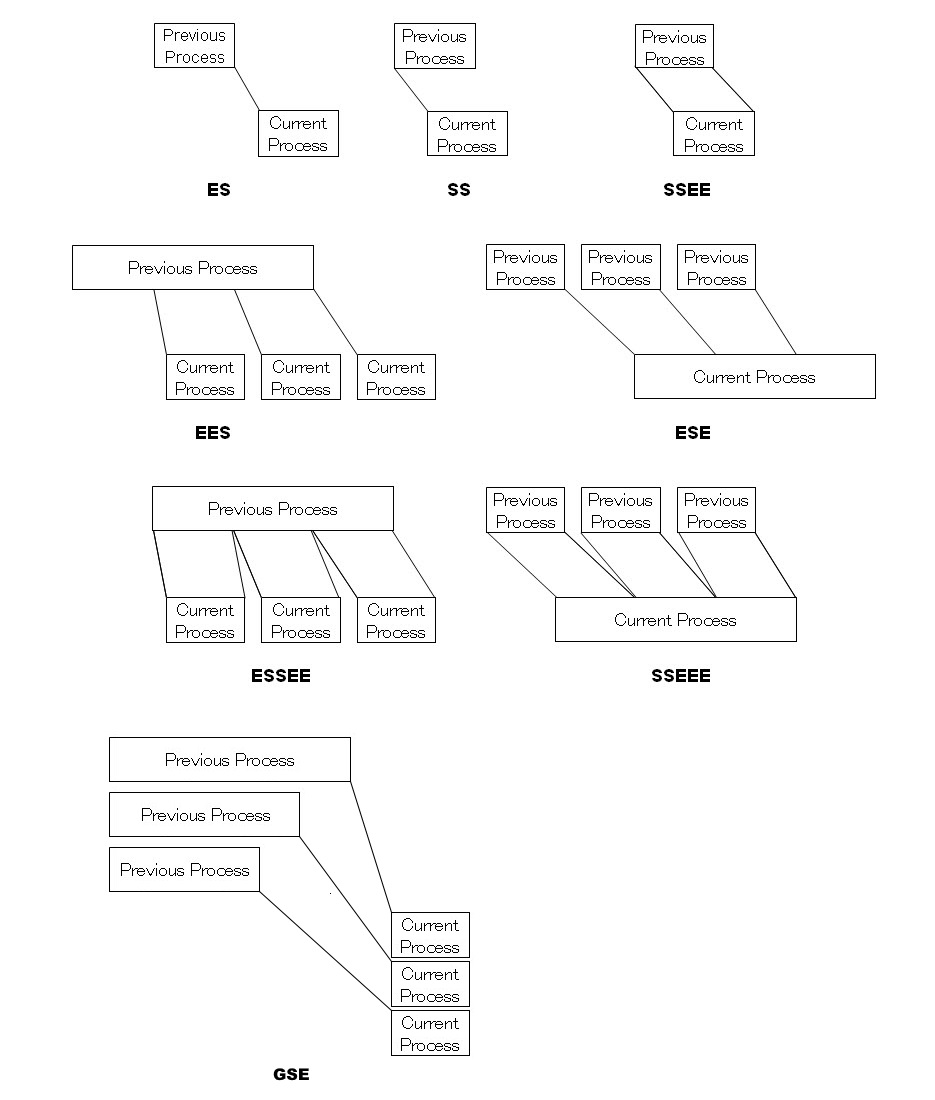Cases when you use ESE, EES, SSEEE, ESSEE and operations aren't split

When the Time constraint method is ESE and the previous process is not split then as shown below the time relationship is the same as ES.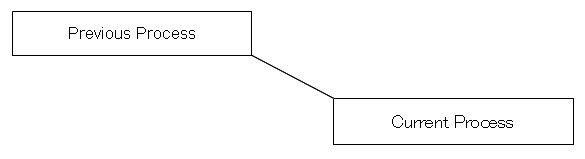When the Time constraint method is EES and the next process is not split then as shown below the time relationship is the same as ES.When the Time constraint method is SSEEE and the previous process is not split then as shown below the time relationship is the same as SSEE.When the Time constraint method is ESSEE and the next process is not split then as shown below the time relationship is the same as SSEE.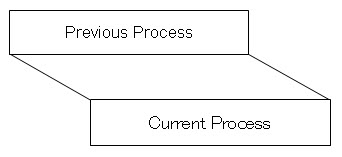Way to definite Time constraint method and Time constraint MIN

Time constraint method and Time constraint MIN can be determined by Master input instruction or Master use instruction in the Integrated Master Editor table.
If they were set in more than one instructions, Time constraint method and Time constraint MIN will be determined by the following rules.

Master input instruction's Time constraint method and Time constraint MIN.

1)If Time constraint method and Time constraint MIN were set in Master input instruction, settings in Master use instructions will be ignored.

2)Time constraint method and Time constraint MIN of Master input instruction can have default values. If Time constraint method and Time constraint MIN were set default values in Master input instruction, settings in Master use instructions will be ignored.

3)If Time constraint method and Time constraint MIN were set in more than one Master input instructions, the constraint time to the previous process operations can be defined by different pegged input instructions.

Master use instruction's Time constraint method and Time constraint MIN.

Basically, Time constraint method and Time constraint MIN on the main resource will be referred. In the complex situation, they will be determined by the following rules.

4)Firstly, if a task's input instruction pegged to previous process exists and the task's use instruction has a use instruction for main resource, time constraint will be decided by its Master use instruction.

5)If the use instruction for main resource does not exist like (1), the use instruction for sub resource whose Time constraint method and Time constraint MIN has been set will be chosen.

6)If (2) does not exist, the one whose time constraint min has been set will be chosen. In this situation, if the input instruction's time constraint method has been set, it will be chosen, if not, the default value ES will be chosen.

*This feature has effected from Ver.11.0.0.42. Please refer to the release note for details.

* How to define time constraint MAX

Time constraint MAX can also be determined by Master input instruction or Master use instruction in the Integrated Master Editor table.
If they are set in more than one instruction, the setting of the instruction that Time constraint MIN is applied to is used.

An example is given below. The following data is based on sample K. The process 70 is a merging process in which there are multiple input instructions.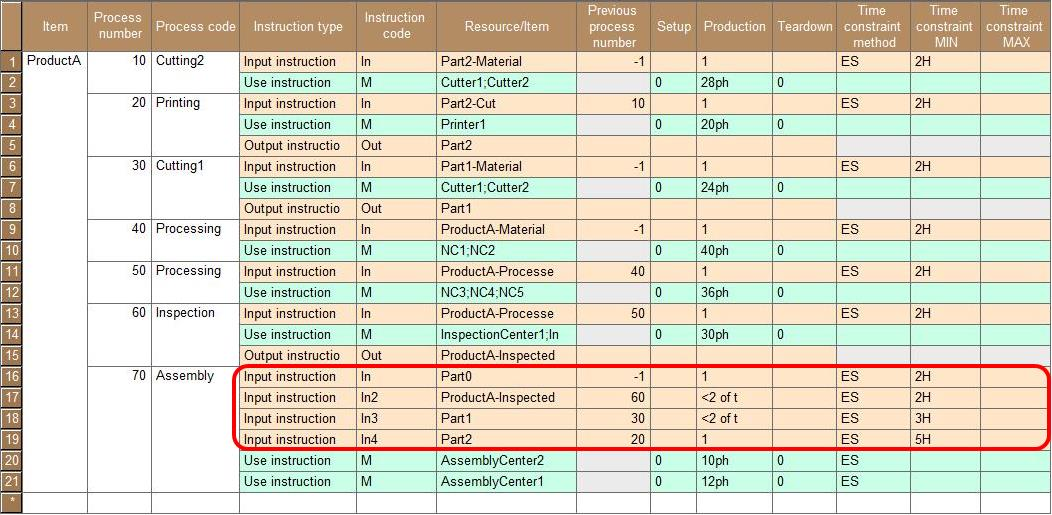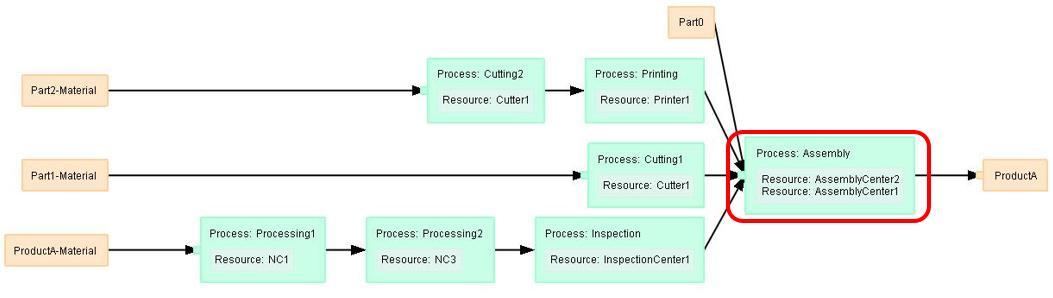As in the following settings, if Time constraint MIN is set for all input instructions, Time constraint MAX can be set for each input instruction.
In the following case, Time constraint MAX is 3 hours between the process 30 and process 7 In this condition, even if you set Time constraint MAX in the use instruction, it will be ignored.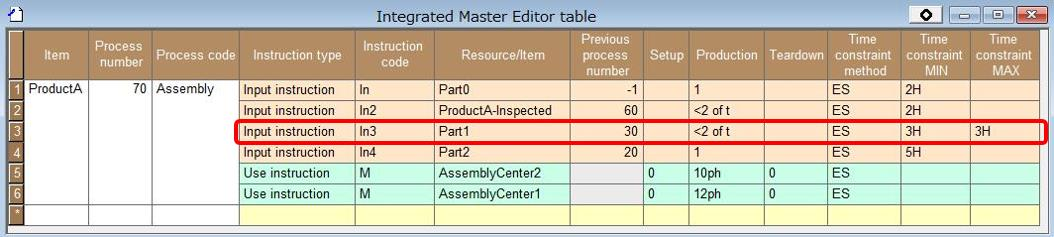In the following example, Time constraint MIN is not set for the input instruction that defines the connection to the process 20. Instead, Time constraint MIN and Time constraint MAX are set for the use instruction.
In such a case, the time constraint between the process 20 and process 70 depends on the use instruction. For other input instructions, time constraint MIN is set. Thus, Time constraint MIN and Time constraint MAX for the use instructions are not applied.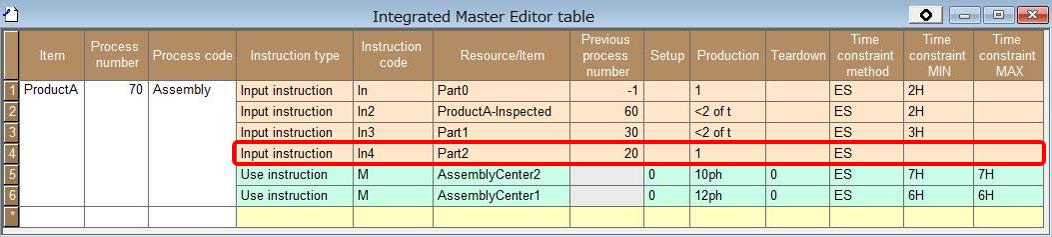*This feature has effected from Ver.12.0.0.14.

Start point of time constraints

For two consecutive processes related according to a time constraint method, tasks for which you enter the input-output ratio in one of the fields Setup, Production, and Teardown of the output instruction of the previous process and the input instruction of the next process will be related.

When the time constraint method is ES, production tasks of two processes will be related by default.This is because the input-output ratio is specified in the Production field of the output instruction of the previous process and of the input instruction of the next process.
* If the entry of the input-output ratio is omitted or the output instruction is omitted, the input-output ratio is regarded to be set in the Production field as "1".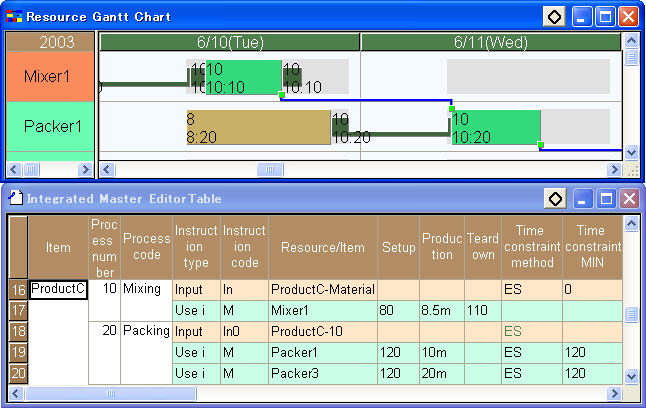When the time constraint method is ES and the input-output ratio is entered in the Setup field of the input instruction of the next process, the end of the production in the previous process and the start of the setup of the next process will be related.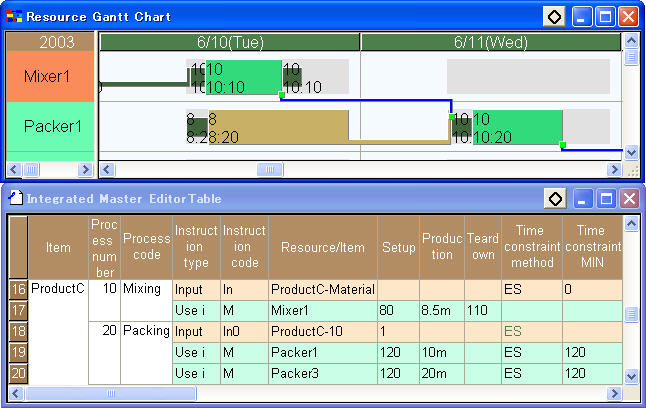When the time constraint method is SSEE and the input-output ratio is entered in the Setup field of the input instruction of the next process, the start and end of the production in the previous process and the start and end of the setup of the next process will be related.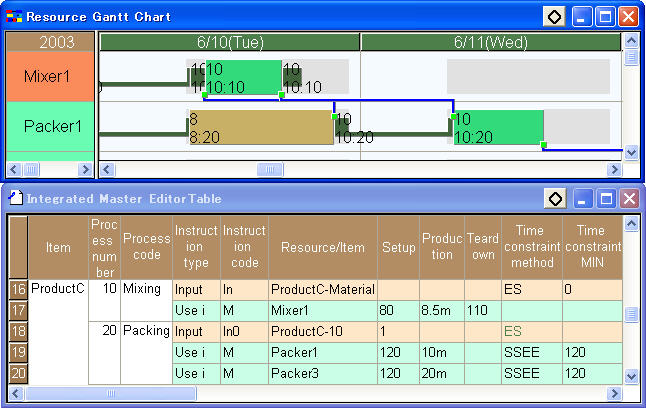When the time constraint method is SSEE and the input-output ratio is entered in the Setup field of the input instruction of the next process and also "C" is specified for the time constraint MIN, the start and end of the production in the previous process will be related to the start of the setup and the end of the production in the next process. Only in this case, one task in the previous process will be related to two tasks in the next process.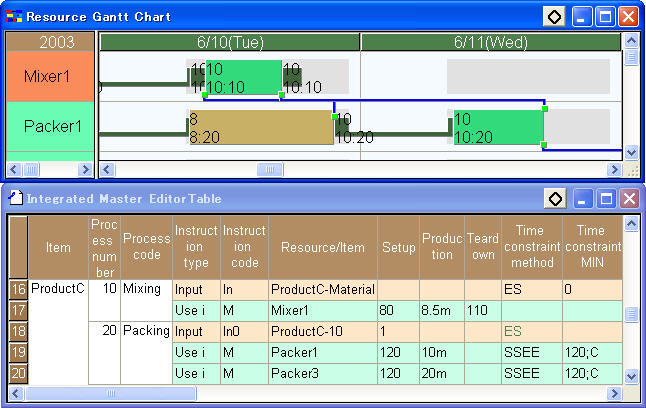Split operations and time constraint method

Among the time constraint methods,

EES,ESE,ESSEE,SSEEE

Cannot correctly make assignments unless the subject operations are already split at the time of making assignments.

Therefore, when splitting by the production unit of resource / master use instruction (cases in which operations are split dynamically while being assigned at the same time)

The following cases will be supported:

The previous process is split by being assigned forward.

ESE,SSEEE

The next process is split by being assigned backward.

EES,ESSEE

The following cases will not be supported:

The next process is split by being assigned forward.

EES,ESSEE

The previous process is split by being assigned backward.

ESE,SSEEE

Those cases where splitting is made prior to assignment (e.g., production unit of items or split number of operations) can be supported as in the cases in which splitting is not made.

* If you enter the input-output ratio in the Production field while you specify "C" in the Time constraint MIN field, the behavior will be unstable.